# scatter

•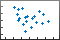## Syntax

``scatter(x,y)``
``scatter(x,y,sz)``
``scatter(x,y,sz,c)``
``scatter(___,"filled")``
``scatter(___,mkr)``
``scatter(tbl,xvar,yvar)``
``scatter(tbl,xvar,yvar,"filled")``
``scatter(ax,___)``
``scatter(___,Name,Value)``
``s = scatter(___)``

## Description

### Vector and Matrix Data

example

````scatter(x,y)` creates a scatter plot with circular markers at the locations specified by the vectors `x` and `y`. To plot one set of coordinates, specify `x` and `y` as vectors of equal length.To plot multiple sets of coordinates on the same set of axes, specify at least one of `x` or `y` as a matrix. ```

example

````scatter(x,y,sz)` specifies the circle sizes. To use the same size for all the circles, specify `sz` as a scalar. To plot each circle with a different size, specify `sz` as a vector or a matrix.```

example

````scatter(x,y,sz,c)` specifies the circle colors. You can specify one color for all the circles, or you can vary the color. For example, you can plot all red circles by specifying `c` as `"red"`.```

example

````scatter(___,"filled")` fills in the circles. Use the `"filled"` option with any of the input argument combinations in the previous syntaxes.```

example

````scatter(___,mkr)` specifies the marker type.```

### Table Data

example

````scatter(tbl,xvar,yvar)` plots the variables `xvar` and `yvar` from the table `tbl`. To plot one data set, specify one variable for `xvar` and one variable for `yvar`. To plot multiple data sets, specify multiple variables for `xvar`, `yvar`, or both. If both arguments specify multiple variables, they must specify the same number of variables. (Since R2021b)```

example

````scatter(tbl,xvar,yvar,"filled")` plots the specified variables from the table with filled circles. (Since R2021b)```

example

````scatter(ax,___)` plots into the axes specified by `ax` instead of into the current axes. The option `ax` can precede any of the input argument combinations in the previous syntaxes.```

example

````scatter(___,Name,Value)` modifies the scatter plot using one or more name-value arguments to set properties. For example: `scatter(x,y,"LineWidth",2)` creates a scatter plot with 2-point marker outlines.`scatter(tbl,"MyX","MyY","ColorVariable","MyColors")` creates a scatter plot from data in a table, and customizes the marker colors using data from the table. For a full list of properties, see Scatter Properties.```

example

````s = scatter(___)` returns the `Scatter` object or an array of `Scatter` objects. Use `s` to set properties after creating the plot. For a full list of properties, see Scatter Properties.```

## Examples

collapse all

Create `x` as 200 equally spaced values between 0 and $3\pi$. Create `y` as cosine values with random noise. Then, create a scatter plot.

```x = linspace(0,3*pi,200); y = cos(x) + rand(1,200); scatter(x,y)```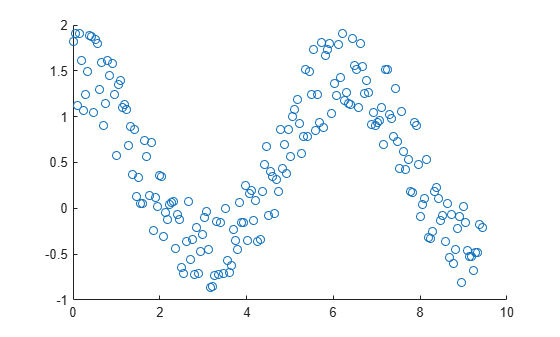Create a scatter plot using circles with different sizes. Specify the size in points squared

```x = linspace(0,3*pi,200); y = cos(x) + rand(1,200); sz = linspace(1,100,200); scatter(x,y,sz)```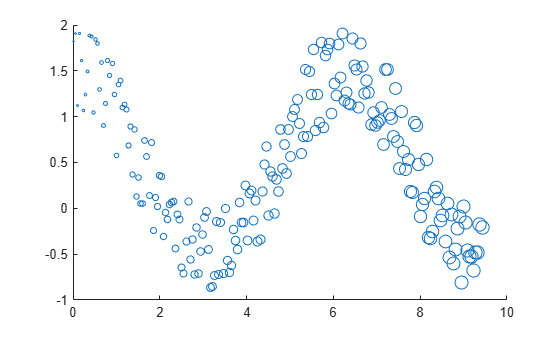Corresponding elements in `x`, `y`, and `sz` determine the location and size of each circle. To plot all circles with the equal area, specify `sz` as a numeric scalar.

Create a scatter plot and vary the circle color.

```x = linspace(0,3*pi,200); y = cos(x) + rand(1,200); c = linspace(1,10,length(x)); scatter(x,y,[],c)```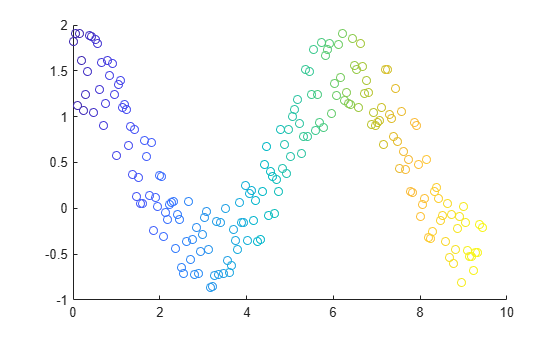Corresponding elements in `x`, `y`, and `c` determine the location and color of each circle. The `scatter` function maps the elements in `c` to colors in the current colormap.

Create a scatter plot and fill in the markers. `scatter` fills each marker using the color of the marker edge.

```x = linspace(0,3*pi,200); y = cos(x) + rand(1,200); sz = 25; c = linspace(1,10,length(x)); scatter(x,y,sz,c,'filled')```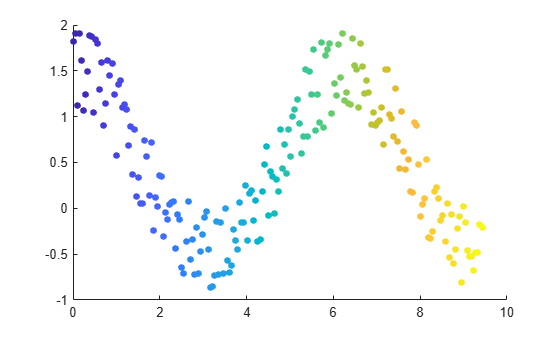Create vectors `x` and `y` as sine and cosine values with random noise. Then, create a scatter plot and use diamond markers with an area of 140 points squared.

```theta = linspace(0,2*pi,150); x = sin(theta) + 0.75*rand(1,150); y = cos(theta) + 0.75*rand(1,150); sz = 140; scatter(x,y,sz,'d')```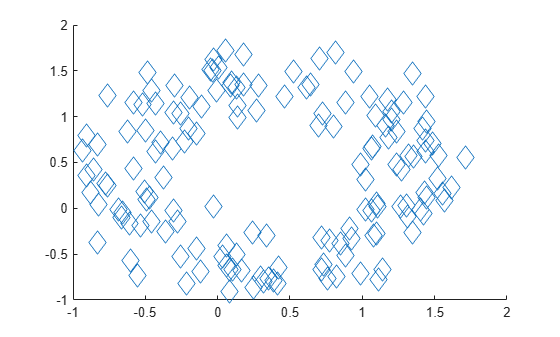Create vectors `x` and `y` as sine and cosine values with random noise. Create a scatter plot and set the marker edge color, marker face color, and line width.

```theta = linspace(0,2*pi,300); x = sin(theta) + 0.75*rand(1,300); y = cos(theta) + 0.75*rand(1,300); sz = 40; scatter(x,y,sz,'MarkerEdgeColor',[0 .5 .5],... 'MarkerFaceColor',[0 .7 .7],... 'LineWidth',1.5)```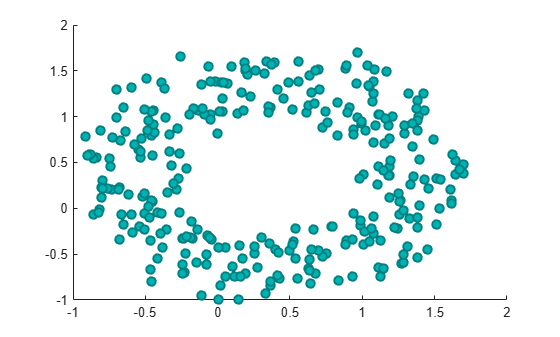You can vary the transparency of scattered points by setting the `AlphaData` property to a vector of different opacity values. To ensure the scatter plot uses the `AlphaData` values, set the `MarkerFaceAlpha` property to `'flat'`.

Create a set of normally distributed random numbers. Then create a scatter plot of the data with filled markers.

```x = randn(1000,1); y = randn(1000,1); s = scatter(x,y,'filled');```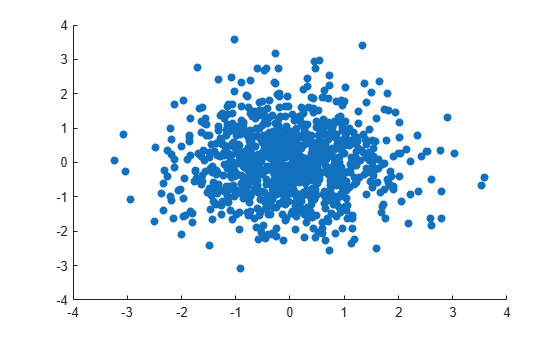Set the opacity of each point according to its distance from zero.

```distfromzero = sqrt(x.^2 + y.^2); s.AlphaData = distfromzero; s.MarkerFaceAlpha = 'flat';```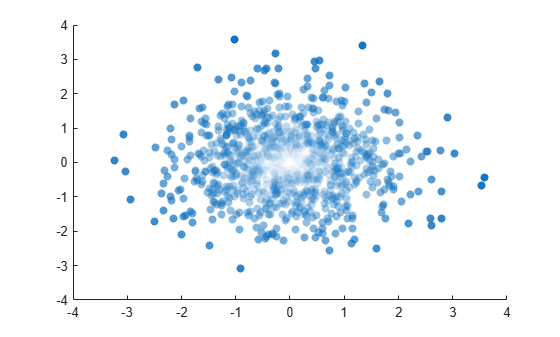Since R2021b

A convenient way to plot data from a table is to pass the table to the `scatter` function and specify the variables you want to plot. For example, read `patients.xls` as a table `tbl`. Plot the relationship between the `Systolic` and `Diastolic` variables by passing `tbl` as the first argument to the `scatter` function followed by the variable names. Notice that the axis labels match the variable names.

```tbl = readtable('patients.xls'); scatter(tbl,'Systolic','Diastolic');```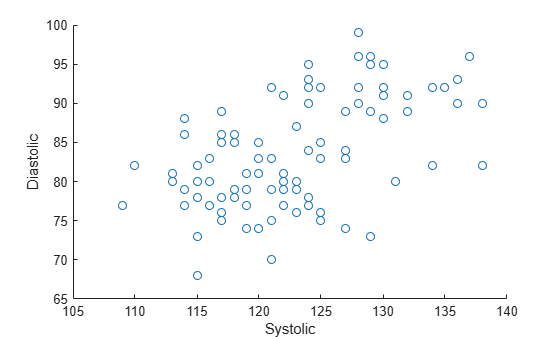You can also plot multiple variables at the same time. For example, plot both blood pressure variables versus the `Weight` variable by specifying the `yvar` argument as the cell array `{'Systolic','Diastolic'}`. Add a legend, and notice that the legend labels match the variable names.

```scatter(tbl,'Weight',{'Systolic','Diastolic'}); legend```Since R2021b

One way to plot data from a table and customize the colors and marker sizes is to set the `ColorVariable` and `SizeData` properties. You can set these properties as name-value arguments when you call the `scatter` function, or you can set them on the `Scatter` object later.

For example, read `patients.xls` as a table `tbl`. Plot the `Height` variable versus the `Weight` variable with filled markers. Vary the marker colors by specifying the `ColorVariable` name-value argument. Return the `Scatter` object as `s`, so you can set other properties later.

```tbl = readtable('patients.xls'); s = scatter(tbl,'Weight','Height','filled','ColorVariable','Diastolic');```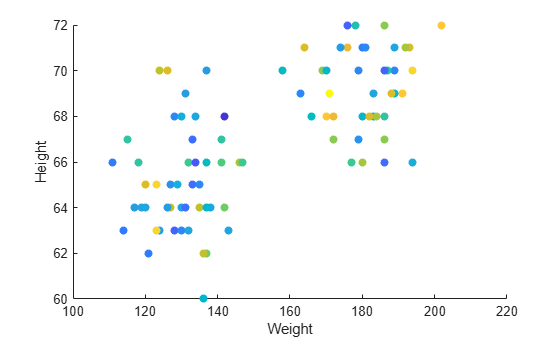Change the marker sizes to `100` points by setting the `SizeData` property. Then add a colorbar.

```s.SizeData = 100; colorbar```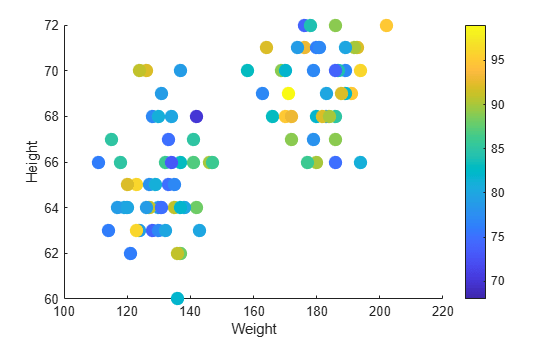Since R2019b

You can display a tiling of plots using the `tiledlayout` and `nexttile` functions. Call the `tiledlayout` function to create a 2-by-1 tiled chart layout. Call the `nexttile` function to create the axes objects `ax1` and `ax2`. Plot scattered data into each axes. In the bottom scatter plot, specify diamond filled diamond markers.

```x = linspace(0,3*pi,200); y = cos(x) + rand(1,200); tiledlayout(2,1) % Top plot ax1 = nexttile; scatter(ax1,x,y) % Bottom plot ax2 = nexttile; scatter(ax2,x,y,'filled','d')```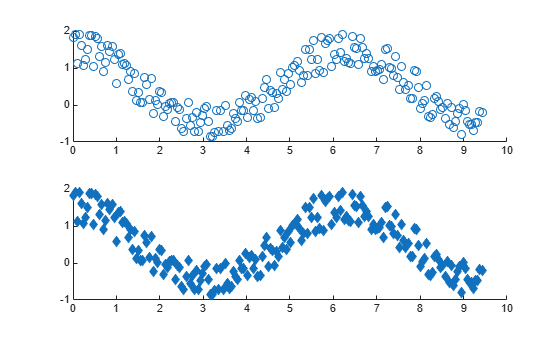Create a scatter plot and return the scatter series object, `s`.

```theta = linspace(0,1,500); x = exp(theta).*sin(100*theta); y = exp(theta).*cos(100*theta); s = scatter(x,y);```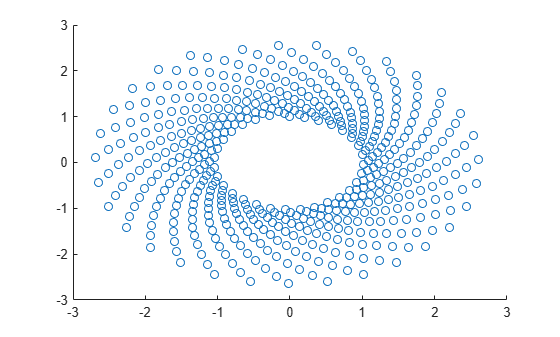Use `s` to query and set properties of the scatter series after it has been created. Set the line width to `0.6` point. Set the marker edge color to blue. Set the marker face color using an RGB triplet color.

```s.LineWidth = 0.6; s.MarkerEdgeColor = 'b'; s.MarkerFaceColor = [0 0.5 0.5];```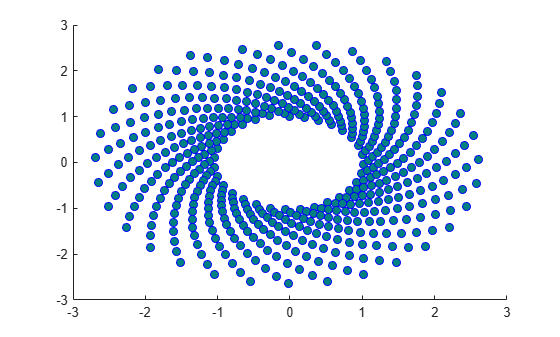## Input Arguments

collapse all

x-coordinates, specified as a scalar, vector, or matrix. The size and shape of `x` depends on the shape of your data. This table describes the most common situations.

Type of PlotHow to Specify Coordinates
Single point

Specify `x` and `y` as scalars. For example:

`scatter(1,2)`

One set of points

Specify `x` and `y` as any combination of row or column vectors of the same length. For example:

`scatter([1 2 3],[4; 5; 6])`

Multiple sets of points that are different colors

If all the sets share the same x- or y-coordinates, specify the shared coordinates as a vector and the other coordinates as a matrix. The length of the vector must match one of the dimensions of the matrix. For example:

`scatter([1 2 3],[4 5 6; 7 8 9])`
If the matrix is square, `scatter` plots a separate set of points for each column in the matrix.

Alternatively, specify `x` and `y` as matrices of equal size. In this case, `scatter` plots each column of `y` against the corresponding column of `x`. For example:

`scatter([1 3 5; 2 4 6],[10 25 45; 20 40 60])`

Data Types: `single` | `double` | `int8` | `int16` | `int32` | `int64` | `uint8` | `uint16` | `uint32` | `uint64` | `categorical` | `datetime` | `duration`

y-coordinates, specified as a scalar, vector, or matrix. The size and shape of `y` depends on the shape of your data. This table describes the most common situations.

Type of PlotHow to Specify Coordinates
Single point

Specify `x` and `y` as scalars. For example:

`scatter(1,2)`

One set of points

Specify `x` and `y` as any combination of row or column vectors of the same length. For example:

`scatter([1 2 3],[4; 5; 6])`

Multiple sets of points that are different colors

If all the sets share the same x- or y-coordinates, specify the shared coordinates as a vector and the other coordinates as a matrix. The length of the vector must match one of the dimensions of the matrix. For example:

`scatter([1 2 3],[4 5 6; 7 8 9])`
If the matrix is square, `scatter` plots a separate set of points for each column in the matrix.

Alternatively, specify `x` and `y` as matrices of equal size. In this case, `scatter` plots each column of `y` against the corresponding column of `x`. For example:

`scatter([1 3 5; 2 4 6],[10 25 45; 20 40 60])`

Data Types: `single` | `double` | `int8` | `int16` | `int32` | `int64` | `uint8` | `uint16` | `uint32` | `uint64` | `categorical` | `datetime` | `duration`

Marker size, specified as a numeric scalar, vector, matrix, or empty array (`[]`). The size controls the area of each marker in points squared. An empty array specifies the default size of 36 points. The way you specify the size depends on how you specify `x` and `y`, and how you want the plot to look. This table describes the most common situations.

Desired Marker Sizes`x` and `y` `sz`Example

Same size for all points

Any valid combination of vectors or matrices described for `x` and `y`.

Scalar

Specify `x` as a vector, `y` as a matrix, and `sz` as a scalar.

```x = [1 2 3 4]; y = [1 6; 3 8; 2 7; 4 9]; scatter(x,y,100)```

Different size for each point

Vectors of the same length

• A vector with the same length as `x` and `y`.

• A matrix with at least one dimension that matches the lengths of `x` and `y`. Specifying a matrix is useful for displaying multiple markers with different sizes at each (x,y) location.

Specify `x`, `y`, and `sz` as vectors.

```x = [1 2 3 4]; y = [1 3 2 4]; sz = [80 150 700 50]; scatter(x,y,sz)```

Specify `x` and `y` as vectors and `sz` as a matrix.

```x = [1 2 3 4]; y = [1 3 2 4]; sz = [80 30; 150 900; 50 2000; 200 350]; scatter(x,y,sz)```

Different size for each point

At least one of `x` or `y` is a matrix for plotting multiple data sets

• A vector with the same number of elements as there are points in each data set.

• A matrix that has the same size as the `x` or `y` matrix.

Specify `x` as a vector, `y` as a matrix, and `sz` as vector.

```x = [1 2 3 4]; y = [1 6; 3 8; 2 7; 4 9]; sz = [80 150 50 700]; scatter(x,y,sz)```

Specify `x` as a vector, `y` as a matrix, and `sz` as a matrix the same size as `y`.

```x = [1 2 3 4]; y = [1 6; 3 8; 2 7; 4 9]; sz = [80 30; 150 900; 50 2000; 200 350]; scatter(x,y,sz)```

Data Types: `single` | `double` | `int8` | `int16` | `int32` | `int64` | `uint8` | `uint16` | `uint32` | `uint64`

Marker color, specified as a color name, RGB triplet, matrix of RGB triplets, or a vector of colormap indices.

• Color name — A color name such as `"red"`, or a short name such as `"r"`.

• RGB triplet — A three-element row vector whose elements specify the intensities of the red, green, and blue components of the color. The intensities must be in the range `[0,1]`; for example, ```[0.4 0.6 0.7]```. RGB triplets are useful for creating custom colors.

• Matrix of RGB triplets — A three-column matrix in which each row is an RGB triplet.

• Vector of colormap indices — A vector of numeric values that is the same length as the `x` and `y` vectors.

The way you specify the color depends on the desired color scheme and whether you are plotting one set of coordinates or multiple sets of coordinates. This table describes the most common situations.

Color SchemeHow to Specify the ColorExample

Use one color for all the points.

Specify a color name or a short name from the table below, or specify one RGB triplet.

Plot one set of points, and specify the color as `"red"`.

`scatter(1:4,[2 5 3 7],[],"red")`

Plot two sets of points, and specify the color as red using an RGB triplet.

`scatter(1:4,[2 5; 1 2; 8 4; 11 9],[],[1 0 0])`

Assign different colors to each point using a colormap.

Specify a row or column vector of numbers. The numbers map into the current colormap array. The smallest value maps to the first row in the colormap, and the largest value maps to the last row. The intermediate values map linearly to the intermediate rows.

If your plot has three points, specify a column vector to ensure the values are interpreted as colormap indices.

You can use this method only when `x`, `y`, and `sz` are all vectors.

Create a vector `c` that specifies four colormap indices. Plot four points using the colors from the current colormap. Then, change the colormap to `winter`.

```c = 1:4; scatter(1:4,[2 5 3 7],[],c) colormap(gca,"winter")```

Create a custom color for each point.

Specify an m-by-3 matrix of RGB triplets, where m is the number of points in the plot.

You can use this method only when `x`, `y`, and `sz` are all vectors.

Create a matrix `c` that specifies RGB triplets for green, red, gray, and purple. Then create a scatter plot of four points using those colors.

```c = [0 1 0; 1 0 0; 0.5 0.5 0.5; 0.6 0 1]; scatter(1:4,[2 5 3 7],[],c) ```

Create a different color for each data set.

Specify an n-by-3 matrix of RGB triplets, where n is the number of data sets.

You can use this method only when at least one of `x`, `y`, or `sz` is a matrix.

Create a matrix `c` that contains two RGB triplets. Then plot two data sets using those colors.

```c = [1 0 0; 0.6 0 1]; s = scatter(1:4,[2 5; 1 2; 8 4; 11 9],[],c) ```

#### Color Names and RGB Triplets for Common Colors

Color NameShort NameRGB TripletHexadecimal Color CodeAppearance
`"red"``"r"``[1 0 0]``"#FF0000"``"green"``"g"``[0 1 0]``"#00FF00"``"blue"``"b"``[0 0 1]``"#0000FF"``"cyan"` `"c"``[0 1 1]``"#00FFFF"``"magenta"``"m"``[1 0 1]``"#FF00FF"``"yellow"``"y"``[1 1 0]``"#FFFF00"``"black"``"k"``[0 0 0]``"#000000"``"white"``"w"``[1 1 1]``"#FFFFFF"`Here are the RGB triplets and hexadecimal color codes for the default colors MATLAB® uses in many types of plots.

`[0 0.4470 0.7410]``"#0072BD"``[0.8500 0.3250 0.0980]``"#D95319"``[0.9290 0.6940 0.1250]``"#EDB120"``[0.4940 0.1840 0.5560]``"#7E2F8E"``[0.4660 0.6740 0.1880]``"#77AC30"``[0.3010 0.7450 0.9330]``"#4DBEEE"``[0.6350 0.0780 0.1840]``"#A2142F"`Marker symbol, specified as one of the values listed in this table.

MarkerDescriptionResulting Marker
`"o"`Circle`"+"`Plus sign`"*"`Asterisk`"."`Point`"x"`Cross`"_"`Horizontal line`"|"`Vertical line`"square"`Square`"diamond"`Diamond`"^"`Upward-pointing triangle`"v"`Downward-pointing triangle`">"`Right-pointing triangle`"<"`Left-pointing triangle`"pentagram"`Pentagram`"hexagram"`HexagramOption to fill the interior of the markers, specified as `"filled"`. Use this option with markers that have a face, for example, `"o"` or `"square"`. Markers that do not have a face and contain only edges do not draw (`"+"`, `"*"`, `"."`, and `"x"`).

The `"filled"` option sets the `MarkerFaceColor` property of the `Scatter` object to `"flat"` and the `MarkerEdgeColor` property to `"none"`, so the marker faces draw, but the edges do not.

Source table containing the data to plot, specified as a table or a timetable.

Table variables containing the x-coordinates, specified as one or more table variable indices.

#### Specifying Table Indices

Use any of the following indexing schemes to specify the desired variable or variables.

Indexing SchemeExamples

Variable names:

• `"A"` or `'A'` — A variable called `A`

• `["A","B"]` or `{'A','B'}` — Two variables called `A` and `B`

• `"Var"+digitsPattern(1)` — Variables named `"Var"` followed by a single digit

Variable index:

• An index number that refers to the location of a variable in the table.

• A vector of numbers.

• A logical vector. Typically, this vector is the same length as the number of variables, but you can omit trailing `0` or `false` values.

• `3` — The third variable from the table

• `[2 3]` — The second and third variables from the table

• `[false false true]` — The third variable

Variable type:

• `vartype("categorical")` — All the variables containing categorical values

The table variables you specify can contain numeric, categorical, datetime, or duration values.

To plot one data set, specify one variable for `xvar`, and one variable for `yvar`. For example, read `Patients.xls` into the table `tbl`. Plot the `Diastolic` variable versus the `Weight` variable.

```tbl = readtable("Patients.xls"); scatter(tbl,"Weight","Diastolic")```

To plot multiple data sets together, specify multiple variables for `xvar`, `yvar`, or both. If you specify multiple variables for both arguments, the number of variables must be the same.

For example, plot the `Systolic` and `Diastolic` variables against the `Weight` variable.

`scatter(tbl,"Weight",["Systolic","Diastolic"])`

You can use different indexing schemes for `xvar` and `yvar`. For example, specify `xvar` as a variable name and `yvar` as an index number.

`scatter(tbl,"Weight",9)`

Table variables containing the y-coordinates, specified as one or more table variable indices.

#### Specifying Table Indices

Use any of the following indexing schemes to specify the desired variable or variables.

Indexing SchemeExamples

Variable names:

• `"A"` or `'A'` — A variable called `A`

• `["A","B"]` or `{'A','B'}` — Two variables called `A` and `B`

• `"Var"+digitsPattern(1)` — Variables named `"Var"` followed by a single digit

Variable index:

• An index number that refers to the location of a variable in the table.

• A vector of numbers.

• A logical vector. Typically, this vector is the same length as the number of variables, but you can omit trailing `0` or `false` values.

• `3` — The third variable from the table

• `[2 3]` — The second and third variables from the table

• `[false false true]` — The third variable

Variable type:

• `vartype("categorical")` — All the variables containing categorical values

The table variables you specify can contain numeric, categorical, datetime, or duration values.

To plot one data set, specify one variable for `xvar`, and one variable for `yvar`. For example, read `Patients.xls` into the table `tbl`. Plot the `Diastolic` variable versus the `Weight` variable.

```tbl = readtable("Patients.xls"); scatter(tbl,"Weight","Diastolic")```

To plot multiple data sets together, specify multiple variables for `xvar`, `yvar`, or both. If you specify multiple variables for both arguments, the number of variables must be the same.

For example, plot the `Systolic` and `Diastolic` variables against the `Weight` variable.

`scatter(tbl,"Weight",["Systolic","Diastolic"])`

You can use different indexing schemes for `xvar` and `yvar`. For example, specify `xvar` as a variable name and `yvar` as an index number.

`scatter(tbl,"Weight",9)`

Target axes, specified as an `Axes` object, a `PolarAxes` object, or a `GeographicAxes` object. If you do not specify the axes and the current axes object is Cartesian, then the `scatter` function plots into the current axes.

A convenient way to create scatter plots in polar or geographic coordinates is to use the `polarscatter` or `geoscatter` functions.

### Name-Value Arguments

Specify optional pairs of arguments as `Name1=Value1,...,NameN=ValueN`, where `Name` is the argument name and `Value` is the corresponding value. Name-value arguments must appear after other arguments, but the order of the pairs does not matter.

Before R2021a, use commas to separate each name and value, and enclose `Name` in quotes.

Example: `"MarkerFaceColor","red"` sets the marker face color to red.

The `Scatter` object properties listed here are only a subset. For a complete list, see Scatter Properties.

Marker outline color, specified `"flat"`, an RGB triplet, a hexadecimal color code, a color name, or a short name. The default value of `"flat"` uses colors from the `CData` property.

For a custom color, specify an RGB triplet or a hexadecimal color code.

• An RGB triplet is a three-element row vector whose elements specify the intensities of the red, green, and blue components of the color. The intensities must be in the range `[0,1]`, for example, ```[0.4 0.6 0.7]```.

• A hexadecimal color code is a string scalar or character vector that starts with a hash symbol (`#`) followed by three or six hexadecimal digits, which can range from `0` to `F`. The values are not case sensitive. Therefore, the color codes `"#FF8800"`, `"#ff8800"`, `"#F80"`, and `"#f80"` are equivalent.

Alternatively, you can specify some common colors by name. This table lists the named color options, the equivalent RGB triplets, and hexadecimal color codes.

Color NameShort NameRGB TripletHexadecimal Color CodeAppearance
`"red"``"r"``[1 0 0]``"#FF0000"``"green"``"g"``[0 1 0]``"#00FF00"``"blue"``"b"``[0 0 1]``"#0000FF"``"cyan"` `"c"``[0 1 1]``"#00FFFF"``"magenta"``"m"``[1 0 1]``"#FF00FF"``"yellow"``"y"``[1 1 0]``"#FFFF00"``"black"``"k"``[0 0 0]``"#000000"``"white"``"w"``[1 1 1]``"#FFFFFF"``"none"`Not applicableNot applicableNot applicableNo color

Here are the RGB triplets and hexadecimal color codes for the default colors MATLAB uses in many types of plots.

`[0 0.4470 0.7410]``"#0072BD"``[0.8500 0.3250 0.0980]``"#D95319"``[0.9290 0.6940 0.1250]``"#EDB120"``[0.4940 0.1840 0.5560]``"#7E2F8E"``[0.4660 0.6740 0.1880]``"#77AC30"``[0.3010 0.7450 0.9330]``"#4DBEEE"``[0.6350 0.0780 0.1840]``"#A2142F"`Example: `[0.5 0.5 0.5]`

Example: `"blue"`

Example: `"#D2F9A7"`

Marker fill color, specified as `"flat"`, `"auto"`, an RGB triplet, a hexadecimal color code, a color name, or a short name. The `"flat"` option uses the `CData` values. The `"auto"` option uses the same color as the `Color` property for the axes.

For a custom color, specify an RGB triplet or a hexadecimal color code.

• An RGB triplet is a three-element row vector whose elements specify the intensities of the red, green, and blue components of the color. The intensities must be in the range `[0,1]`, for example, ```[0.4 0.6 0.7]```.

• A hexadecimal color code is a string scalar or character vector that starts with a hash symbol (`#`) followed by three or six hexadecimal digits, which can range from `0` to `F`. The values are not case sensitive. Therefore, the color codes `"#FF8800"`, `"#ff8800"`, `"#F80"`, and `"#f80"` are equivalent.

Alternatively, you can specify some common colors by name. This table lists the named color options, the equivalent RGB triplets, and hexadecimal color codes.

Color NameShort NameRGB TripletHexadecimal Color CodeAppearance
`"red"``"r"``[1 0 0]``"#FF0000"``"green"``"g"``[0 1 0]``"#00FF00"``"blue"``"b"``[0 0 1]``"#0000FF"``"cyan"` `"c"``[0 1 1]``"#00FFFF"``"magenta"``"m"``[1 0 1]``"#FF00FF"``"yellow"``"y"``[1 1 0]``"#FFFF00"``"black"``"k"``[0 0 0]``"#000000"``"white"``"w"``[1 1 1]``"#FFFFFF"``"none"`Not applicableNot applicableNot applicableNo color

Here are the RGB triplets and hexadecimal color codes for the default colors MATLAB uses in many types of plots.

`[0 0.4470 0.7410]``"#0072BD"``[0.8500 0.3250 0.0980]``"#D95319"``[0.9290 0.6940 0.1250]``"#EDB120"``[0.4940 0.1840 0.5560]``"#7E2F8E"``[0.4660 0.6740 0.1880]``"#77AC30"``[0.3010 0.7450 0.9330]``"#4DBEEE"``[0.6350 0.0780 0.1840]``"#A2142F"`Example: `[0.3 0.2 0.1]`

Example: `"green"`

Example: `"#D2F9A7"`

Width of marker edge, specified as a positive value in point units.

Example: `0.75`

Table variable containing the color data, specified as a variable index into the source table.

#### Specifying the Table Index

Use any of the following indexing schemes to specify the desired variable.

Indexing SchemeExamples

Variable name:

• A string scalar or character vector.

• A `pattern` object. The pattern object must refer to only one variable.

• `"A"` or `'A'` — A variable called `A`

• `"Var"+digitsPattern(1)` — The variable with the name `"Var"` followed by a single digit

Variable index:

• An index number that refers to the location of a variable in the table.

• A logical vector. Typically, this vector is the same length as the number of variables, but you can omit trailing `0` or `false` values.

• `3` — The third variable from the table

• `[false false true]` — The third variable

Variable type:

• A `vartype` subscript that selects a table variable of a specified type. The subscript must refer to only one variable.

• `vartype("double")` — The variable containing double values

#### Specifying Color Data

Specifying the `ColorVariable` property controls the colors of the markers. The data in the variable controls the marker fill color when the `MarkerFaceColor` property is set to `"flat"`. The data can also control the marker outline color, when the `MarkerEdgeColor` is set to `"flat"`.

The table variable you specify can contain values of any numeric type. The values can be in either of the following forms:

• A column of numbers that linearly map into the current colormap.

• A three-column array of RGB triplets. RGB triplets are three-element vectors whose values specify the intensities of the red, green, and blue components of specific colors. The intensities must be in the range `[0,1]`. For example, `[0.5 0.7 1]` specifies a shade of light blue.

When you set the `ColorVariable` property, MATLAB updates the `CData` property.

## Output Arguments

collapse all

`Scatter` object or an array of `Scatter` objects. Use `s` to modify properties of a scatter chart after creating it.

## Version History

Introduced before R2006a

expand all